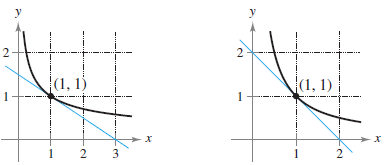×
Get Full Access to Calculus: Early Transcendental Functions - 6 Edition - Chapter 3.2 - Problem 2
Get Full Access to Calculus: Early Transcendental Functions - 6 Edition - Chapter 3.2 - Problem 2

×

# ?Estimating Slope In Exercises 1 and 2, use the graph to estimate the slope of the tangent line to $$y=x^{n}$$ at the point (1, 1). Verify your answerISBN: 9781285774770 141

## Solution for problem 2 Chapter 3.2

Calculus: Early Transcendental Functions | 6th Edition

• Textbook Solutions
• 2901 Step-by-step solutions solved by professors and subject experts
• Get 24/7 help from StudySoup virtual teaching assistantsCalculus: Early Transcendental Functions | 6th Edition

4 5 1 250 Reviews
22
5
Problem 2

Estimating Slope In Exercises 1 and 2, use the graph to estimate the slope of the tangent line to $$y=x^{n}$$ at the point (1, 1). Verify your answer analytically. To print an enlarged copy of the graph, go to MathGraphs.com.

(a) $$y=x^{-1 / 2}$$

(b) $$y=x^-$$Text Transcription:

y=x^n

y=x^-1/2

y=x^-

Step-by-Step Solution:

Step 1 of 5) FOR FURTHER INFORMATIONFor further investigation of solids that have finite volumes and infinite surface areas, see the article “Supersolids: Solids Having Finite Volume and Infinite Surfaces” by William P. Love in Mathematics Teacher. To view this article, go to MathArticles.com.

Step 2 of 2

##### ISBN: 9781285774770

Since the solution to 2 from 3.2 chapter was answered, more than 243 students have viewed the full step-by-step answer. This full solution covers the following key subjects: . This expansive textbook survival guide covers 134 chapters, and 10738 solutions. The full step-by-step solution to problem: 2 from chapter: 3.2 was answered by , our top Calculus solution expert on 11/14/17, 10:53PM. Calculus: Early Transcendental Functions was written by and is associated to the ISBN: 9781285774770. The answer to “?Estimating Slope In Exercises 1 and 2, use the graph to estimate the slope of the tangent line to $$y=x^{n}$$ at the point (1, 1). Verify your answer analytically. To print an enlarged copy of the graph, go to MathGraphs.com.(a) $$y=x^{-1 / 2}$$(b) $$y=x^-$$ Text Transcription:y=x^ny=x^-1/2y=x^-” is broken down into a number of easy to follow steps, and 46 words. This textbook survival guide was created for the textbook: Calculus: Early Transcendental Functions, edition: 6.

## Discover and learn what students are asking

Calculus: Early Transcendental Functions : Functions of Several Variables
?In Exercises 15 - 22, find all first partial derivatives. $$f(x, y)=4 x^{2}-2 x y+y^{2}$$

Statistics: Informed Decisions Using Data : Applications of the Normal Distribution
?Explain why P(X < 30) should be reported as < 60.0001 if X is a normal random variable with mean 100 and standard deviation 15.

Unlock Textbook Solution

?Estimating Slope In Exercises 1 and 2, use the graph to estimate the slope of the tangent line to $$y=x^{n}$$ at the point (1, 1). Verify your answer